# R S Aggarwal Solutions for Class 10 Maths Chapter 12 Trigonometric Ratios of Complementary Angles

R S Aggarwal Class 10 Solutions for Chapter 12 Trigonometric ratios of Complementary Angles, detailed explanations to the questions are available here. This chapter is composed of only one exercise which helps students to solve the trigonometric ratios without using trigonometric tables. Click on the link below to get your R S Aggarwal solutions pdf for free and build your skills.

## Download PDF of R S Aggarwal Solutions for Class 10 Chapter 12 Trigonometric Ratios of Complementary Angles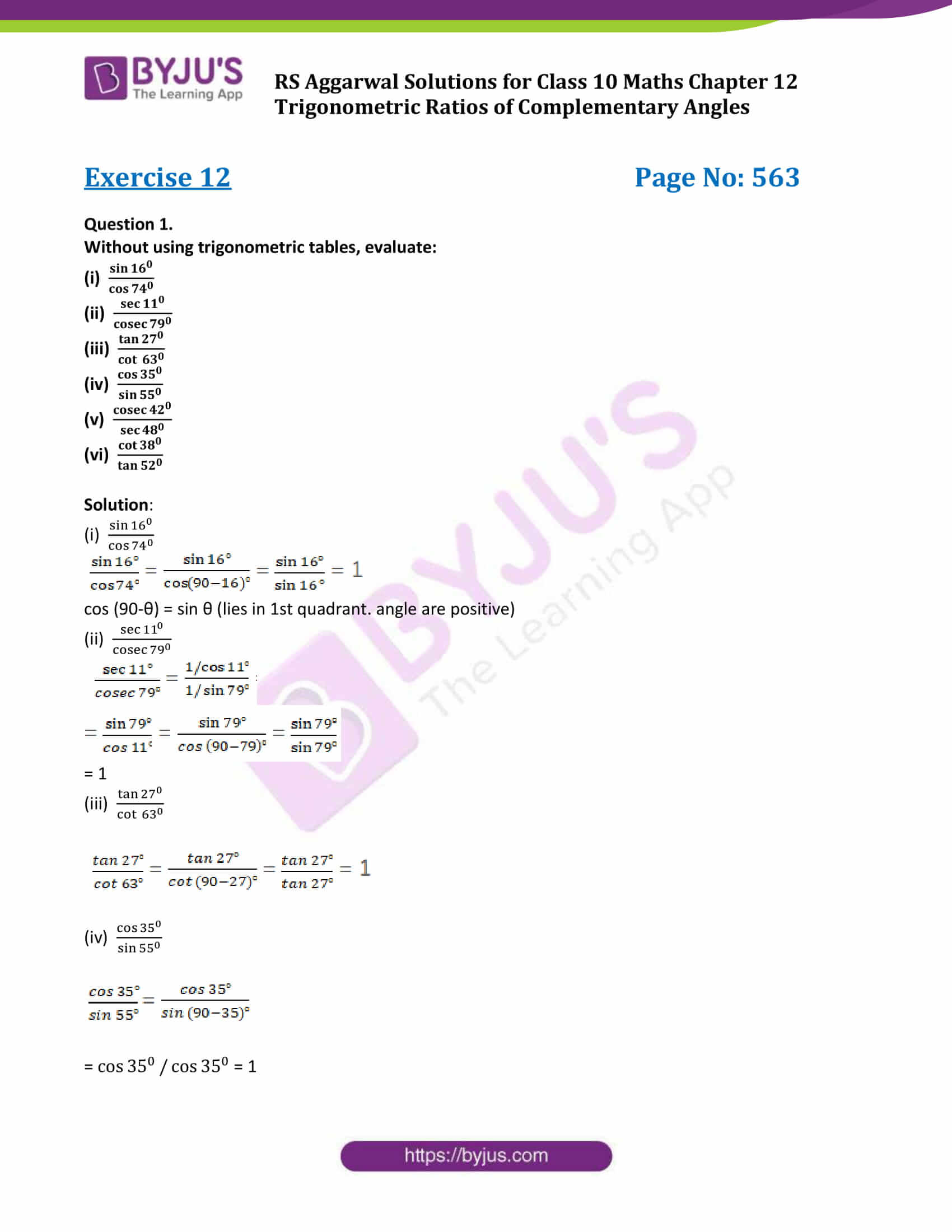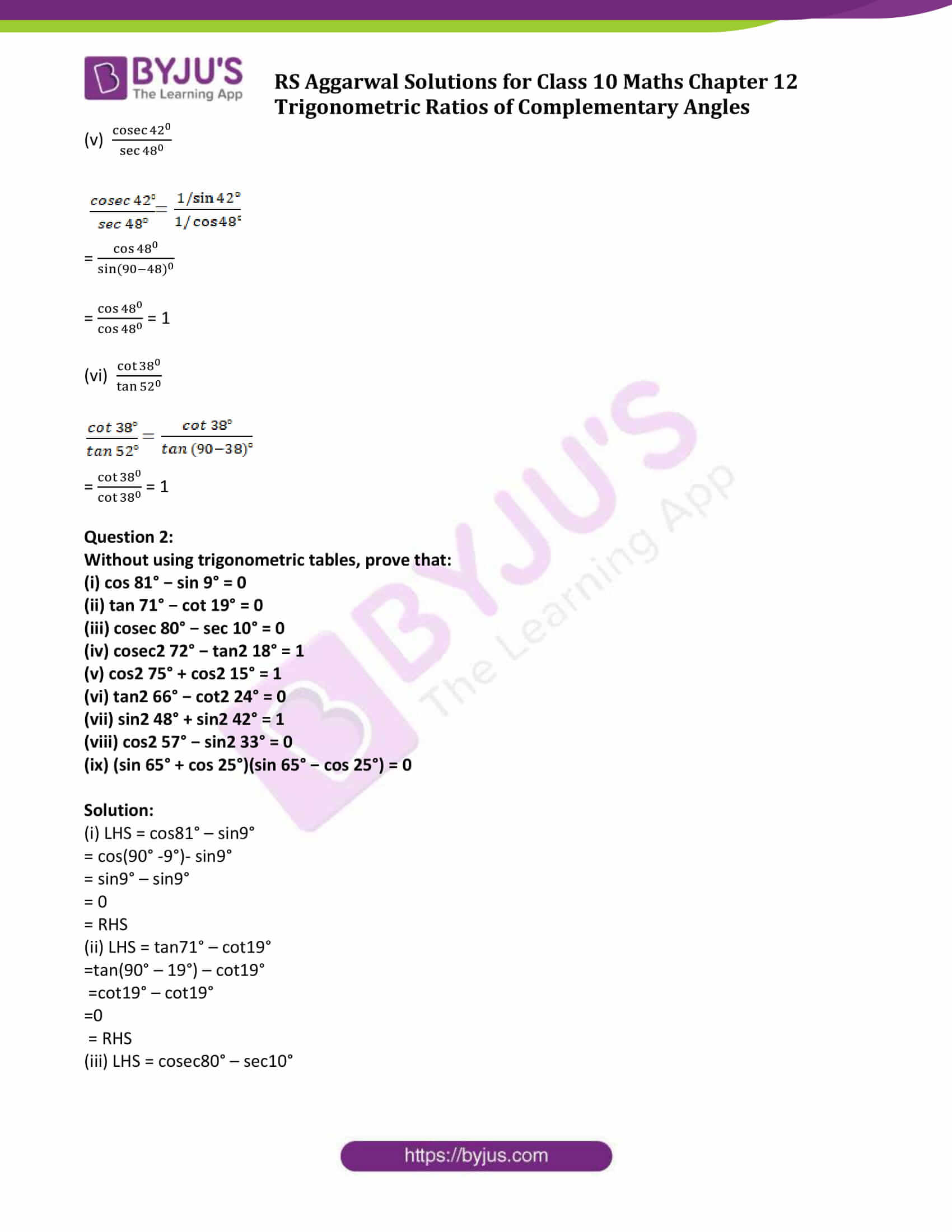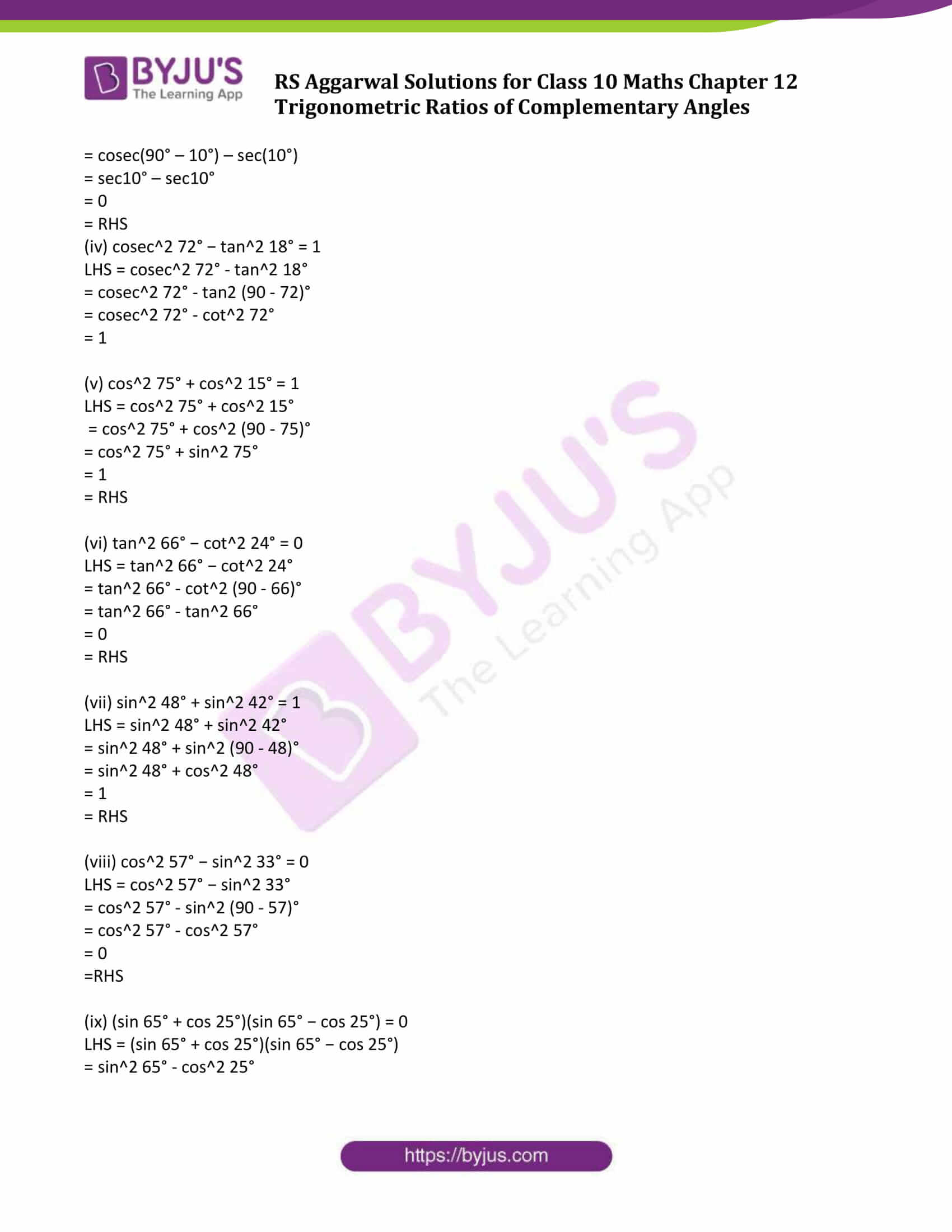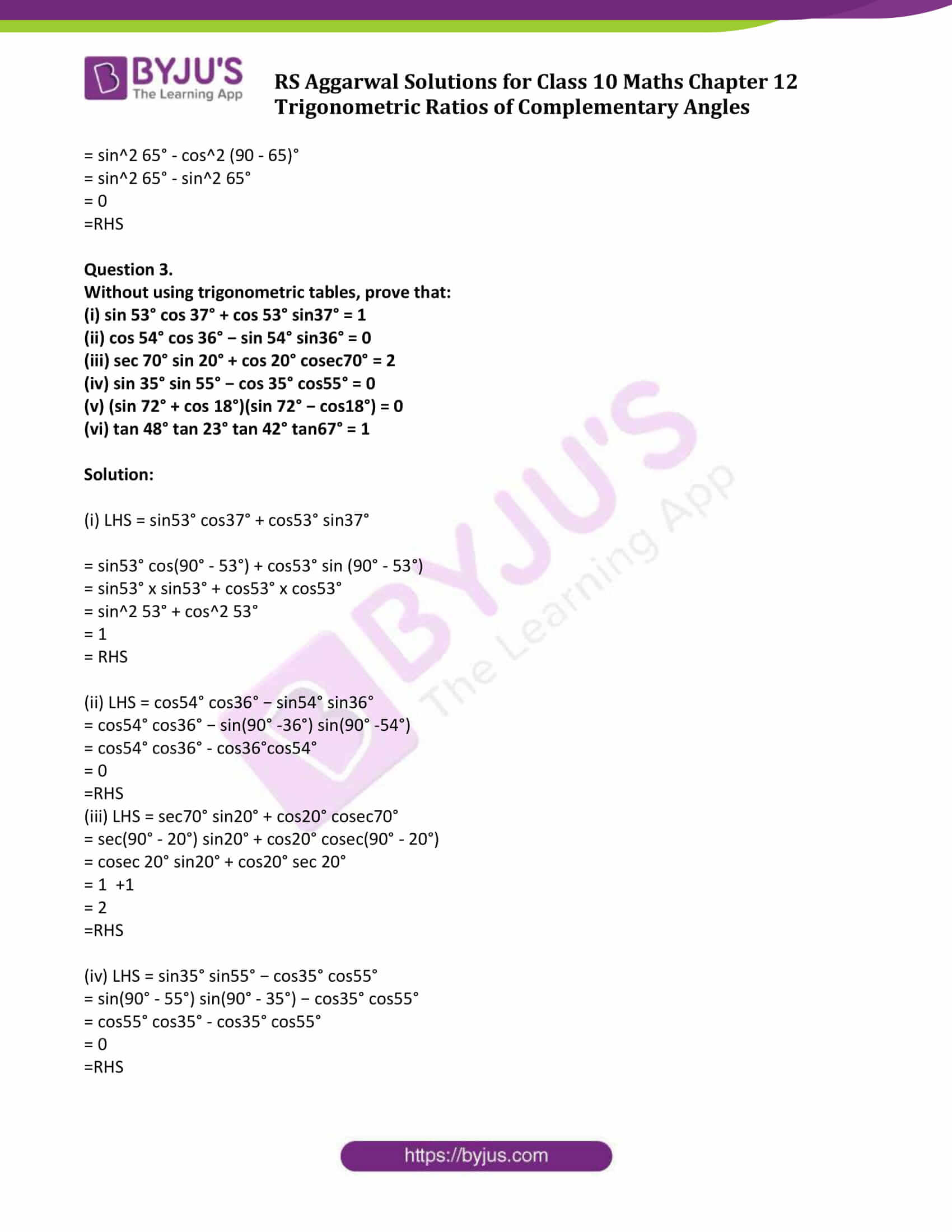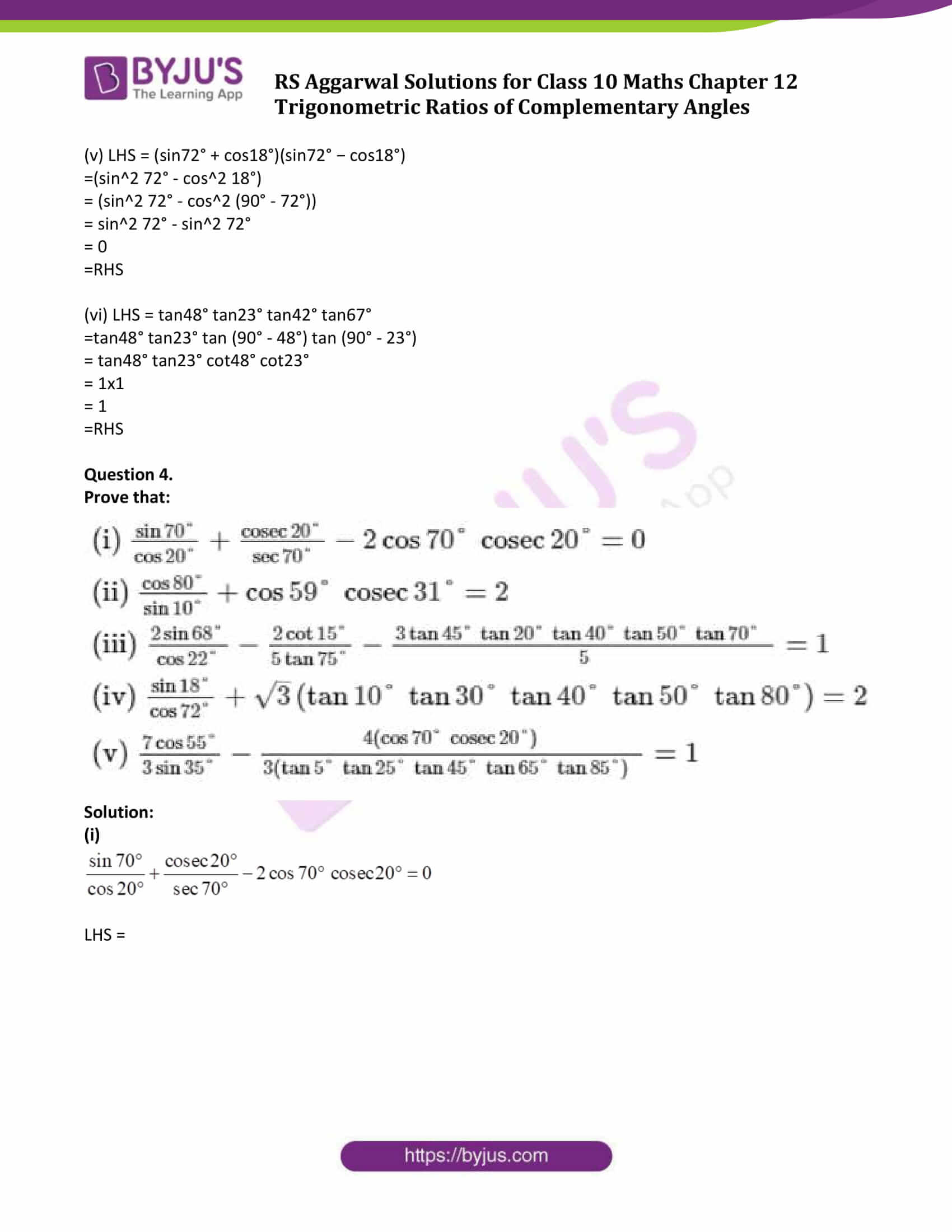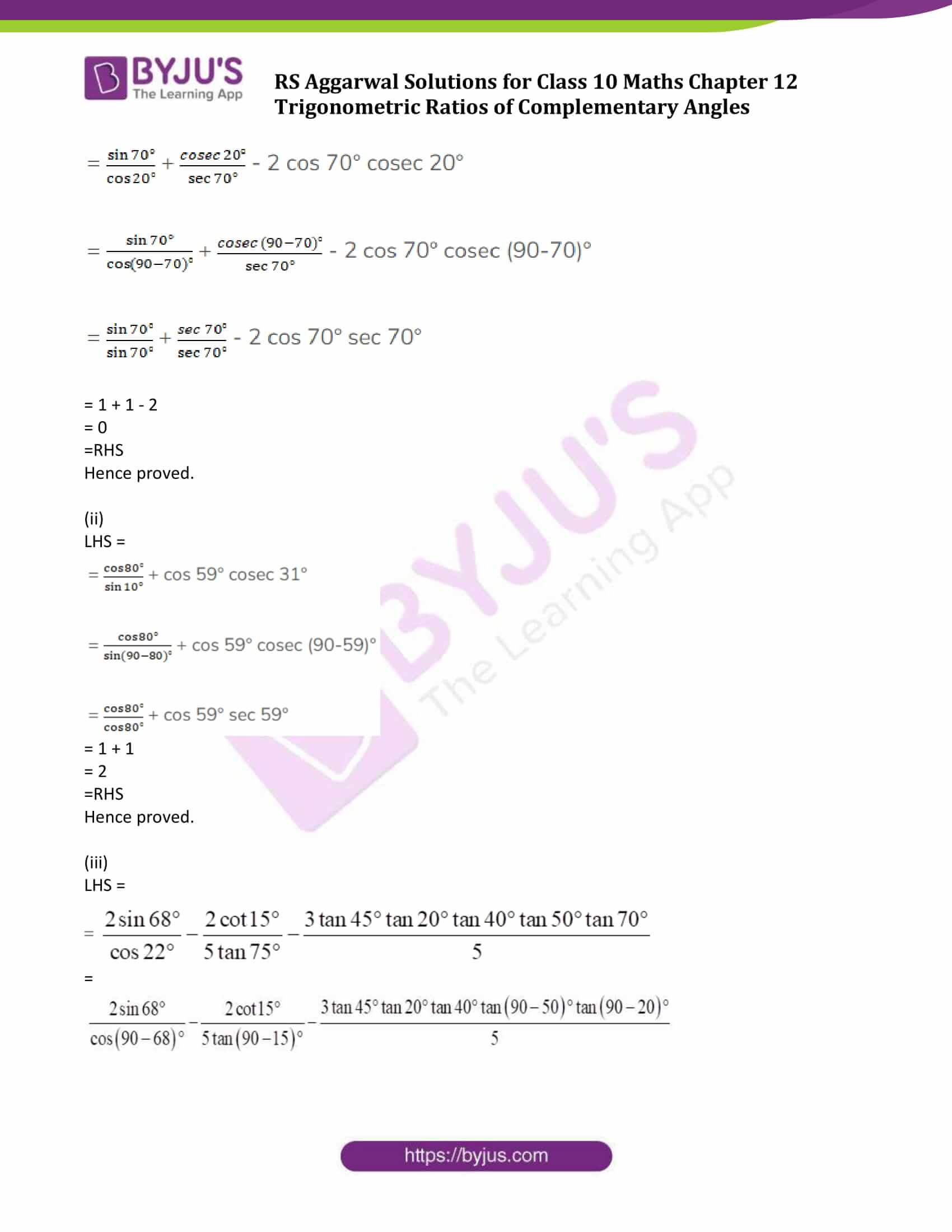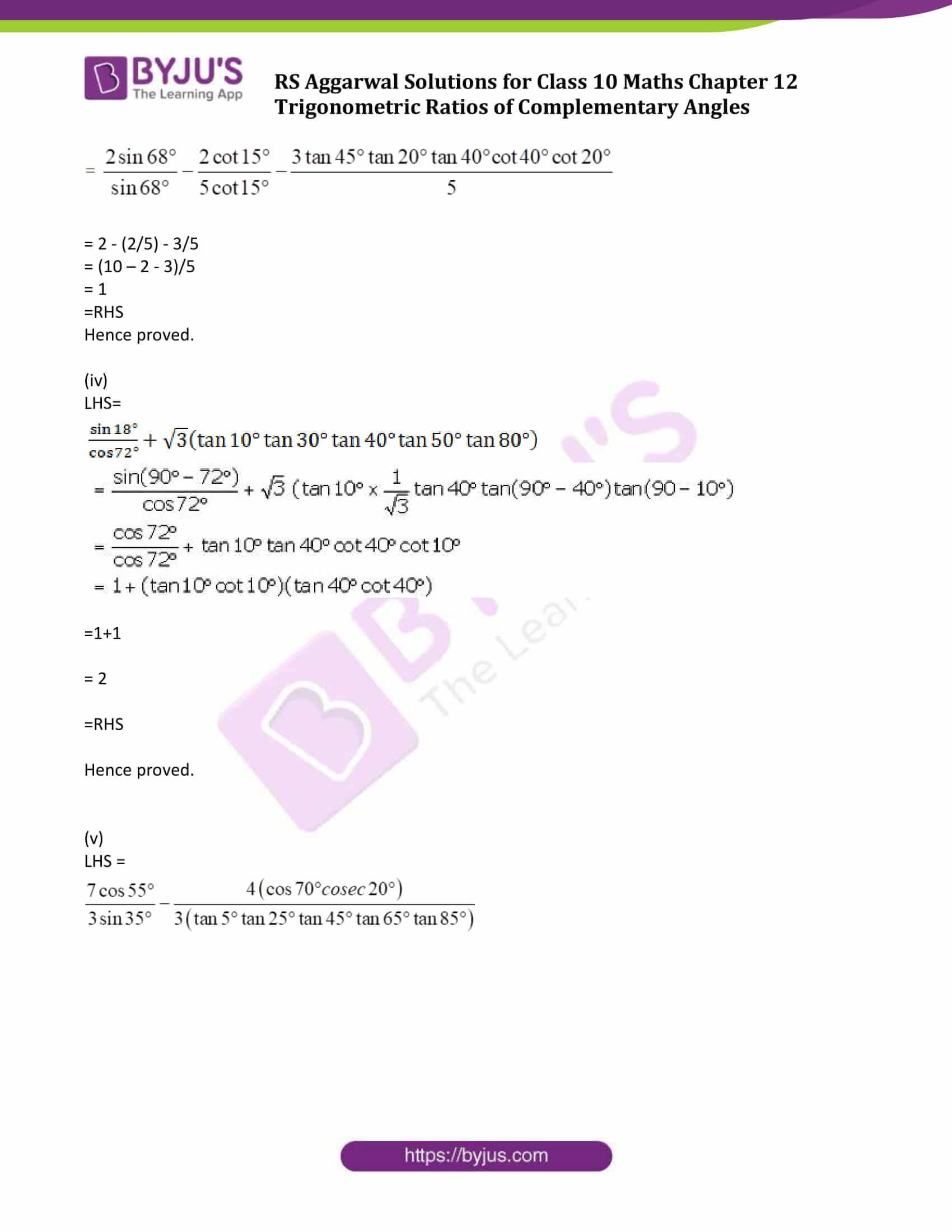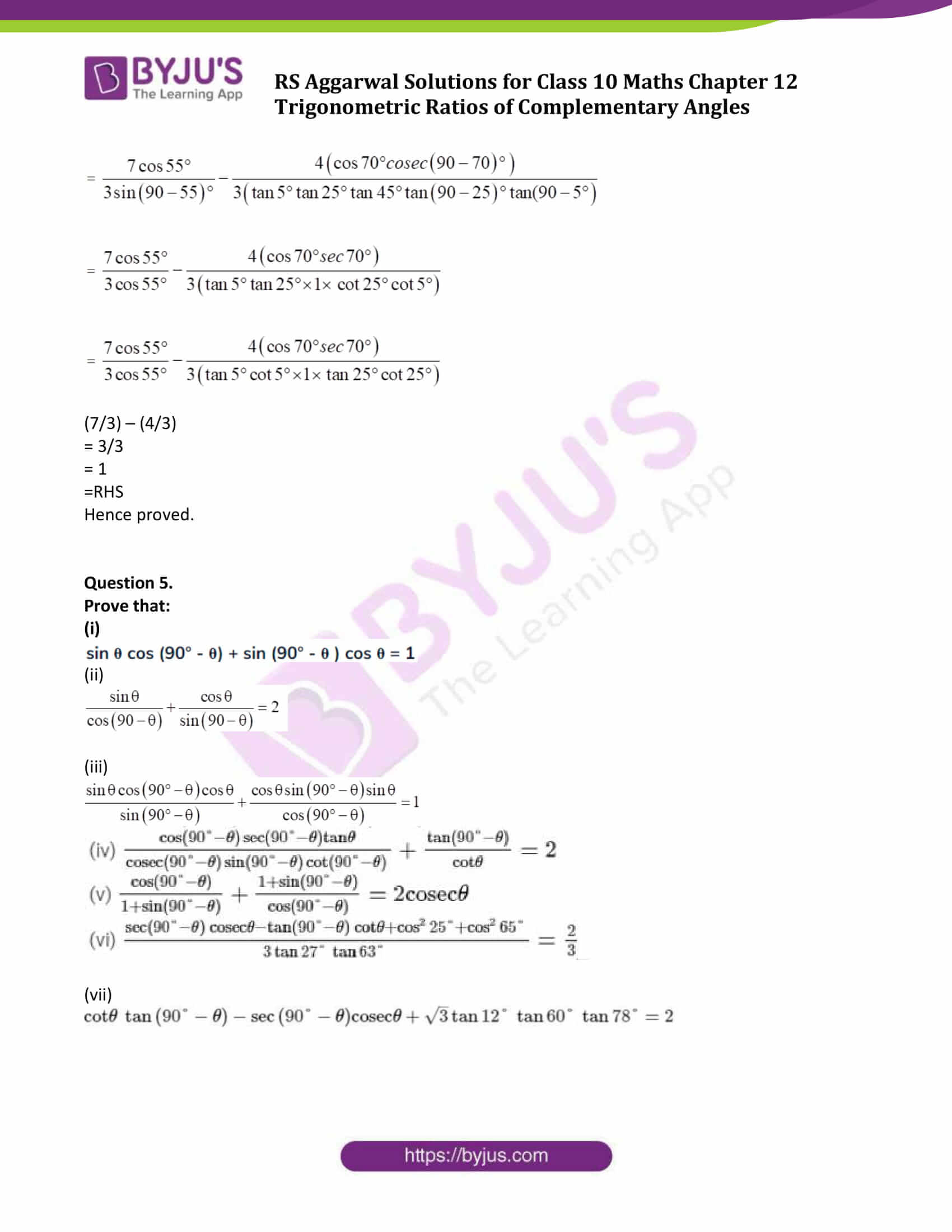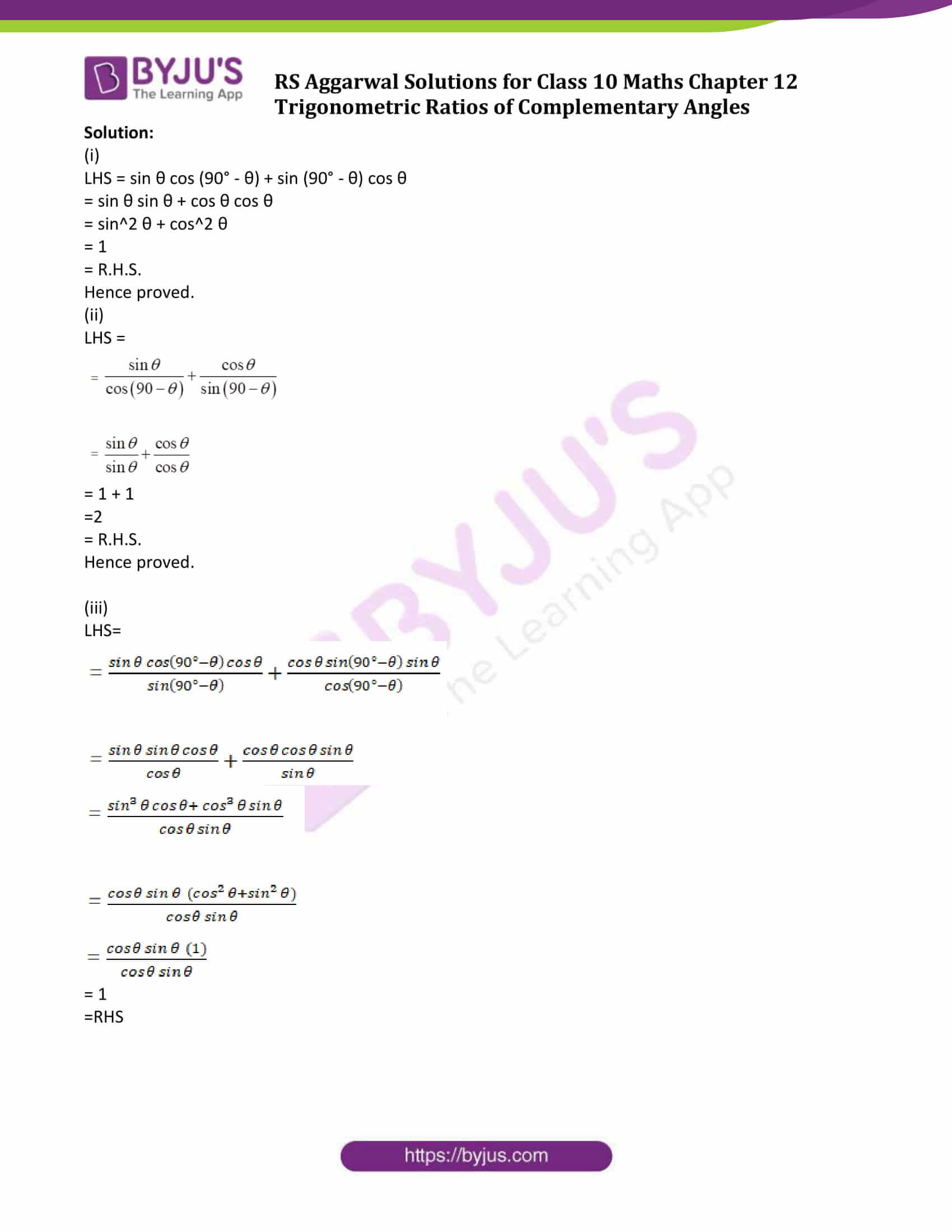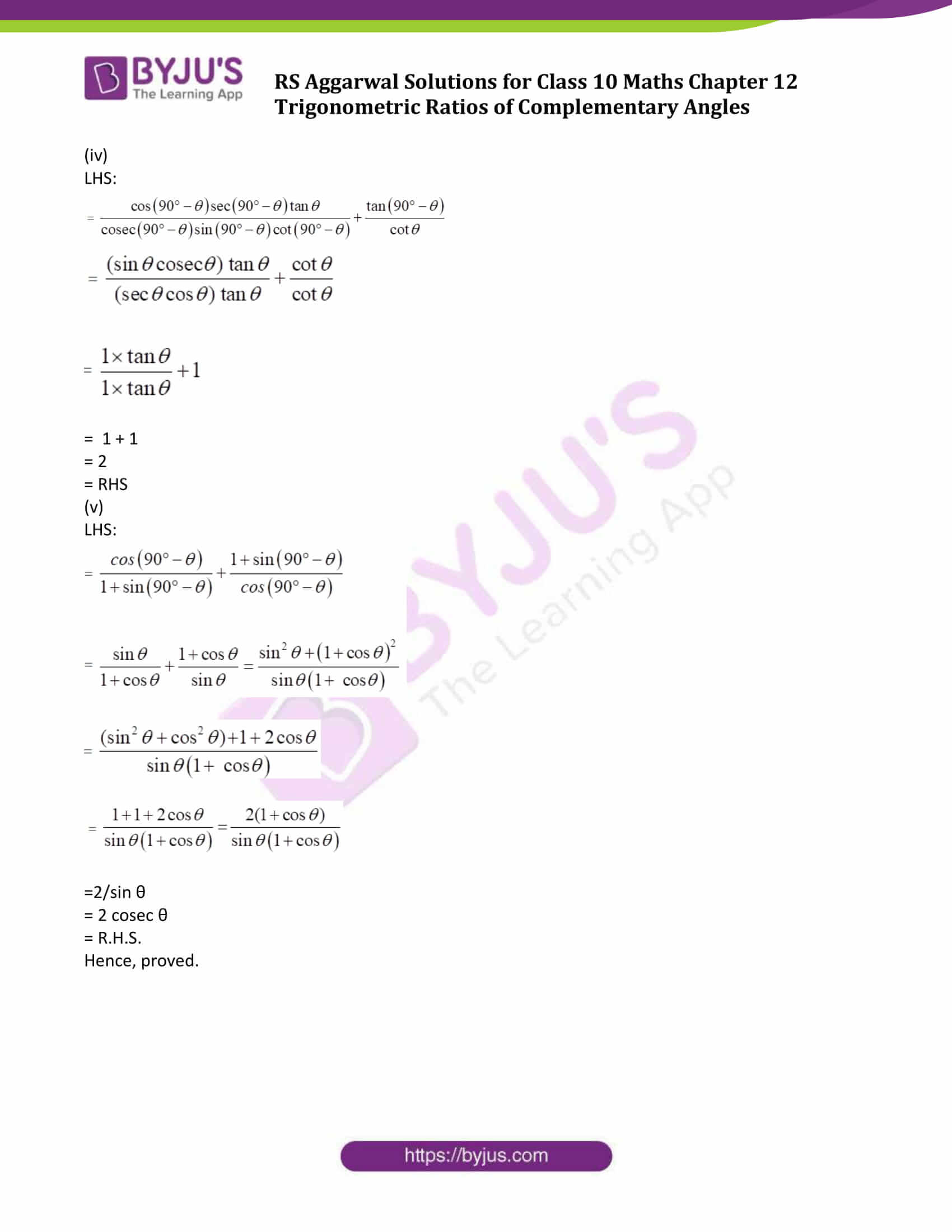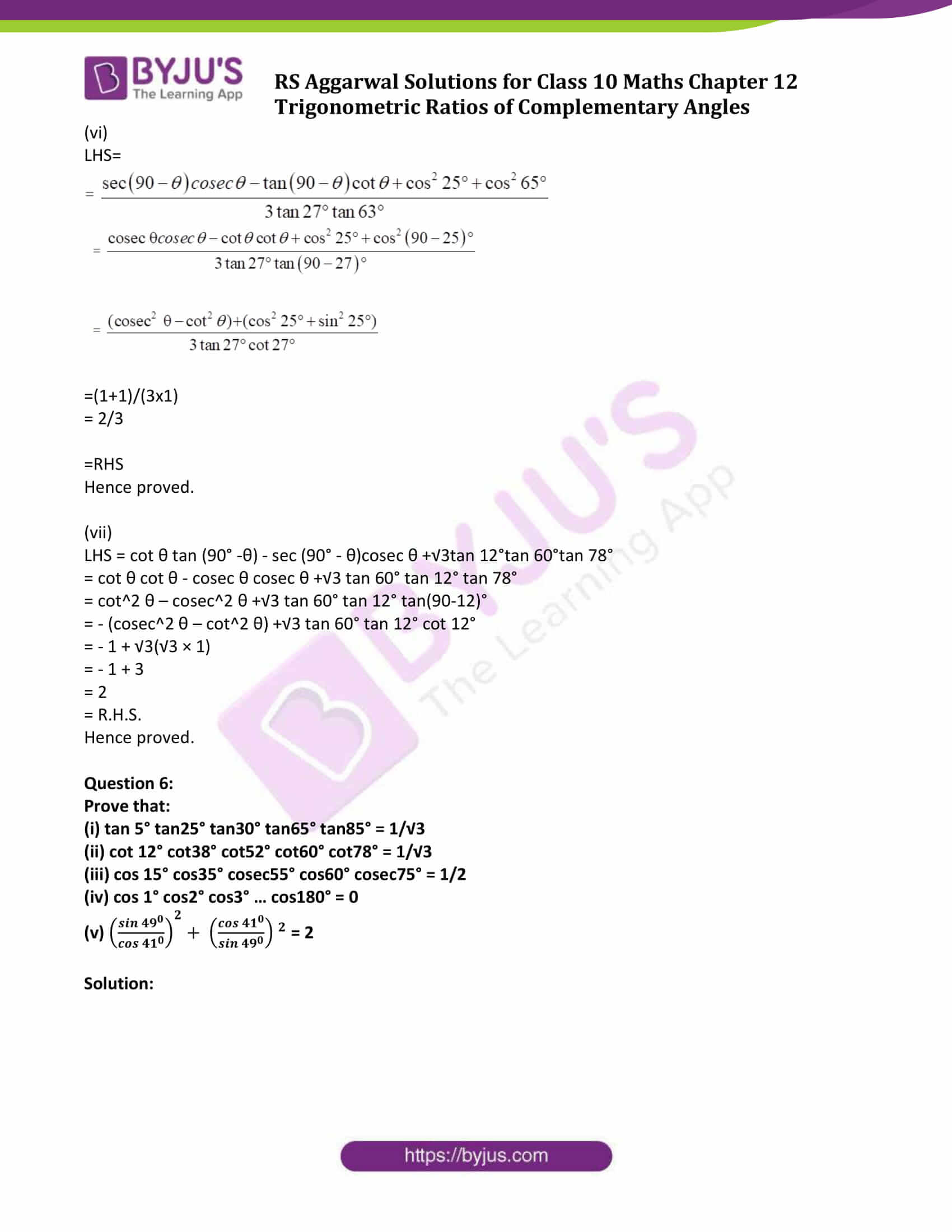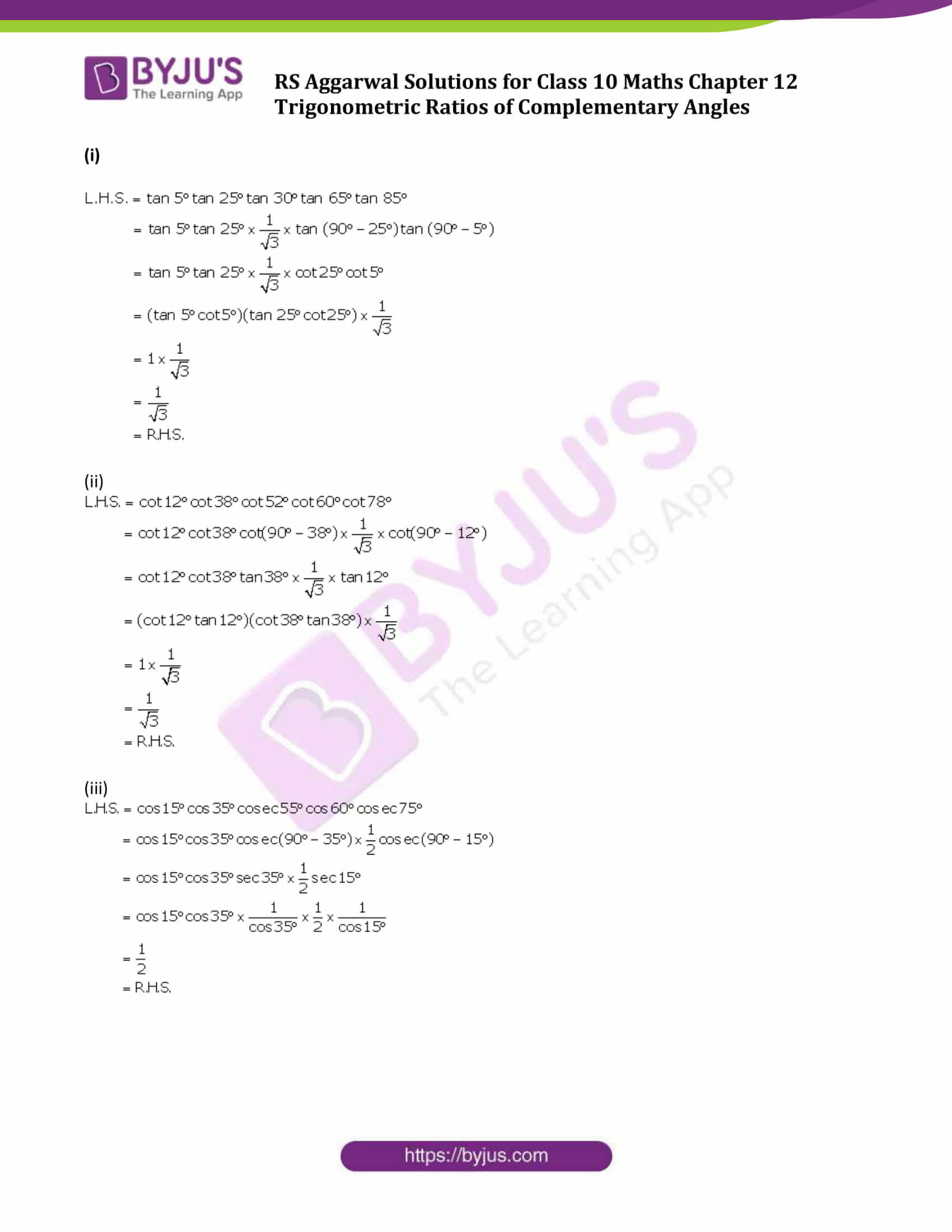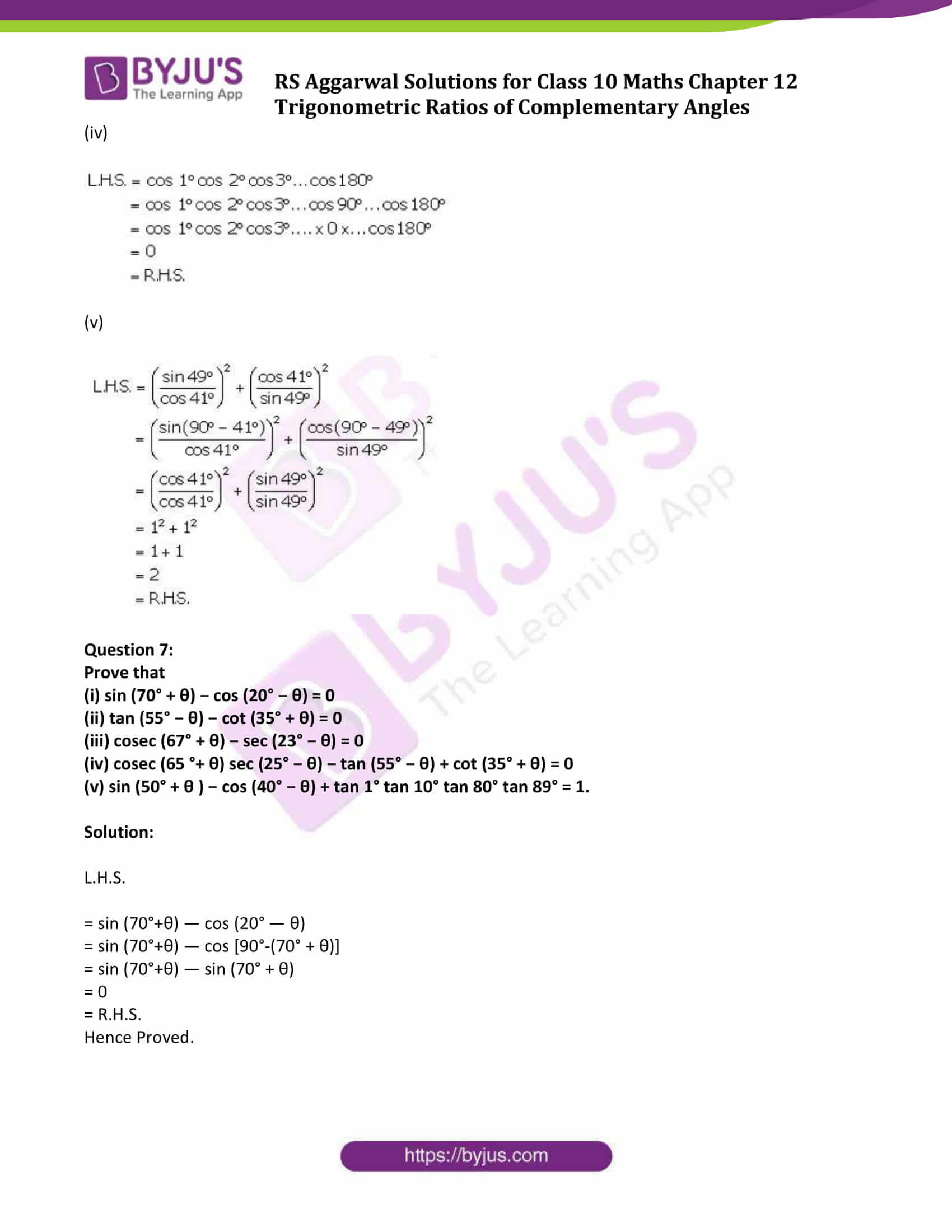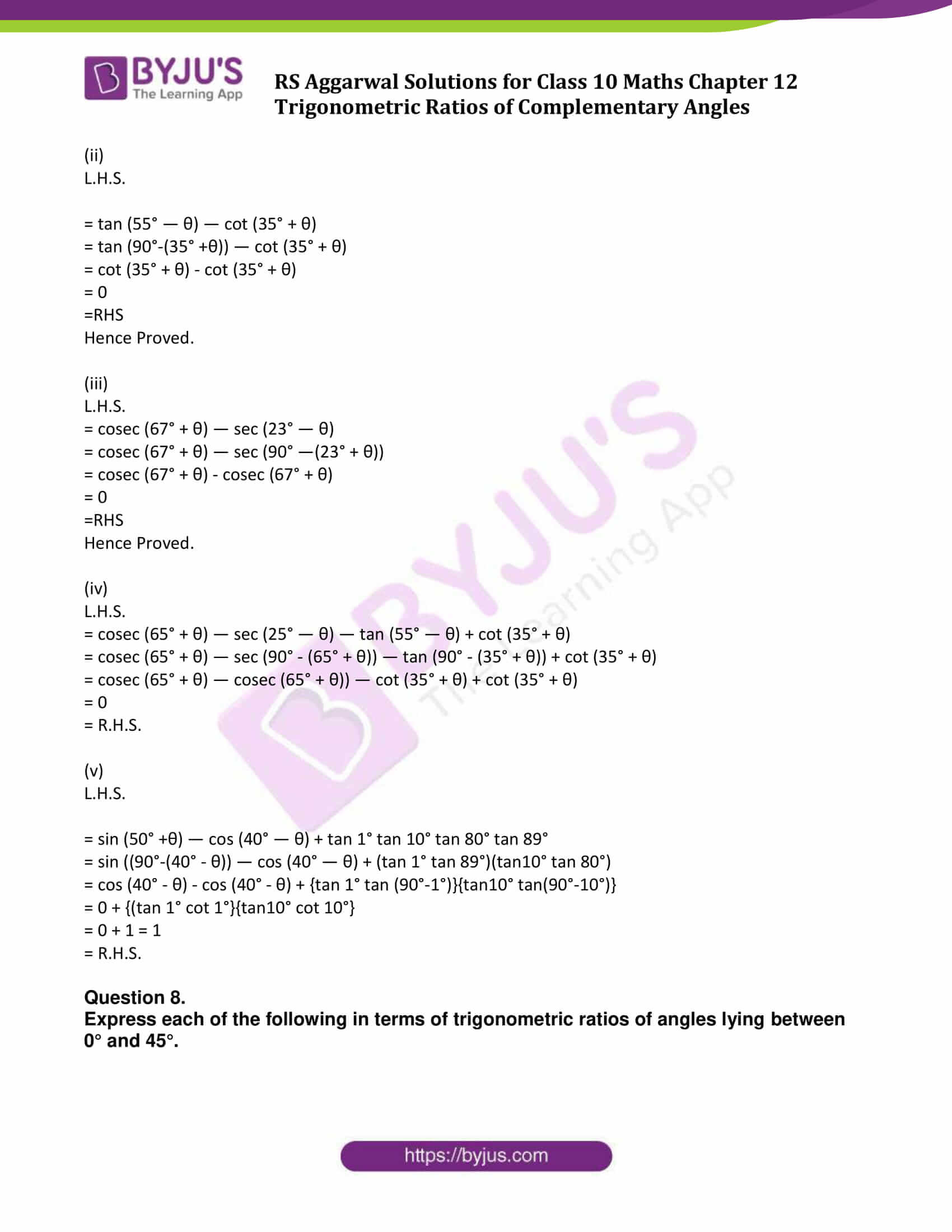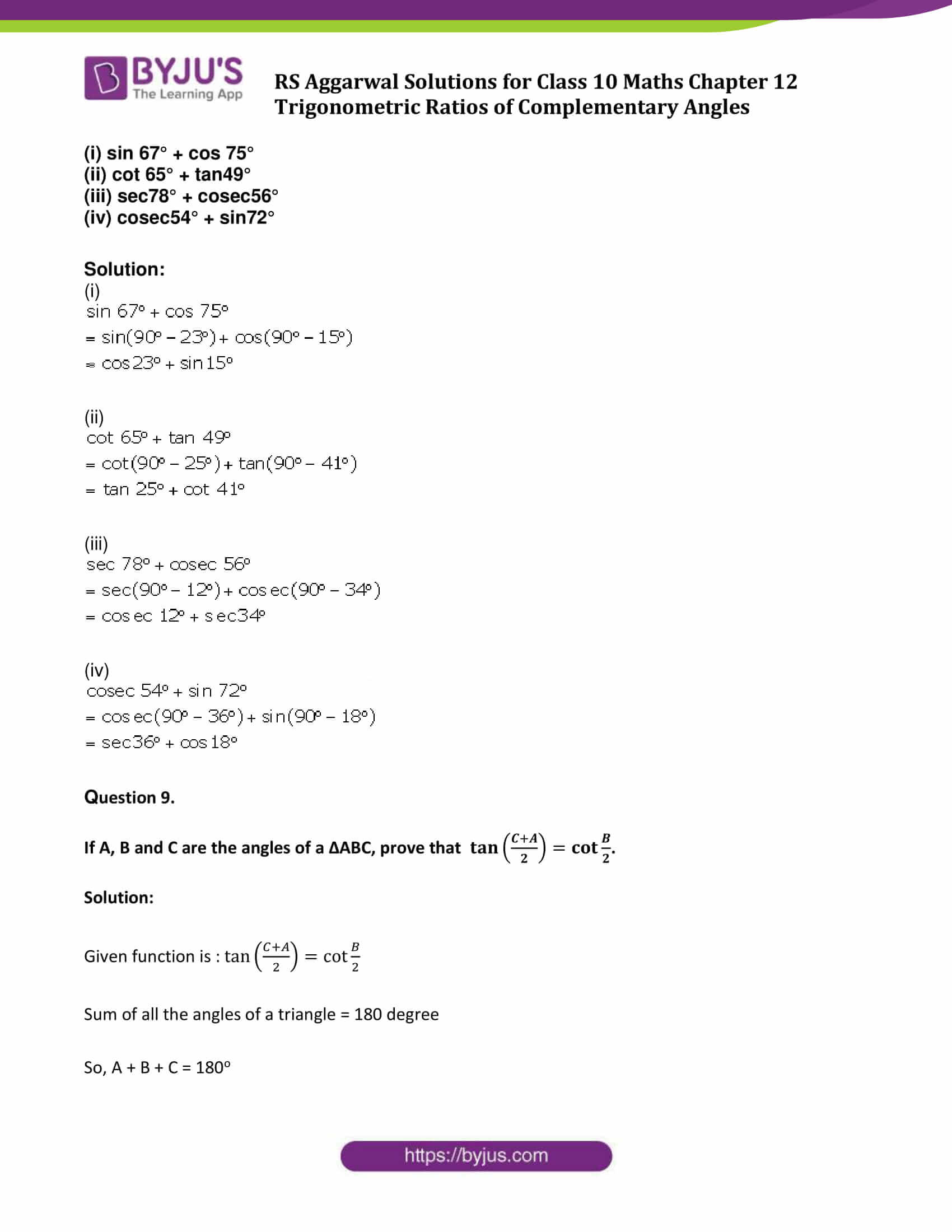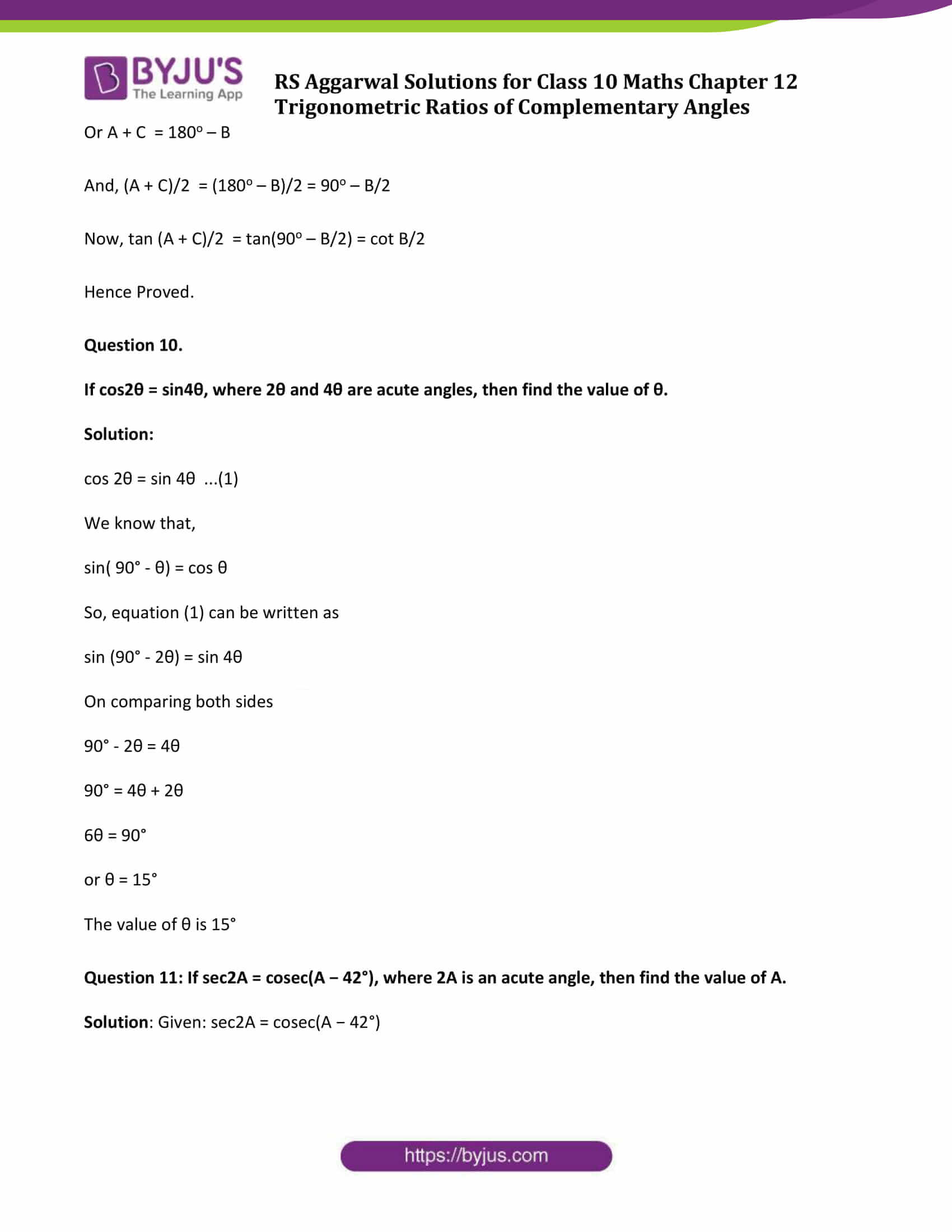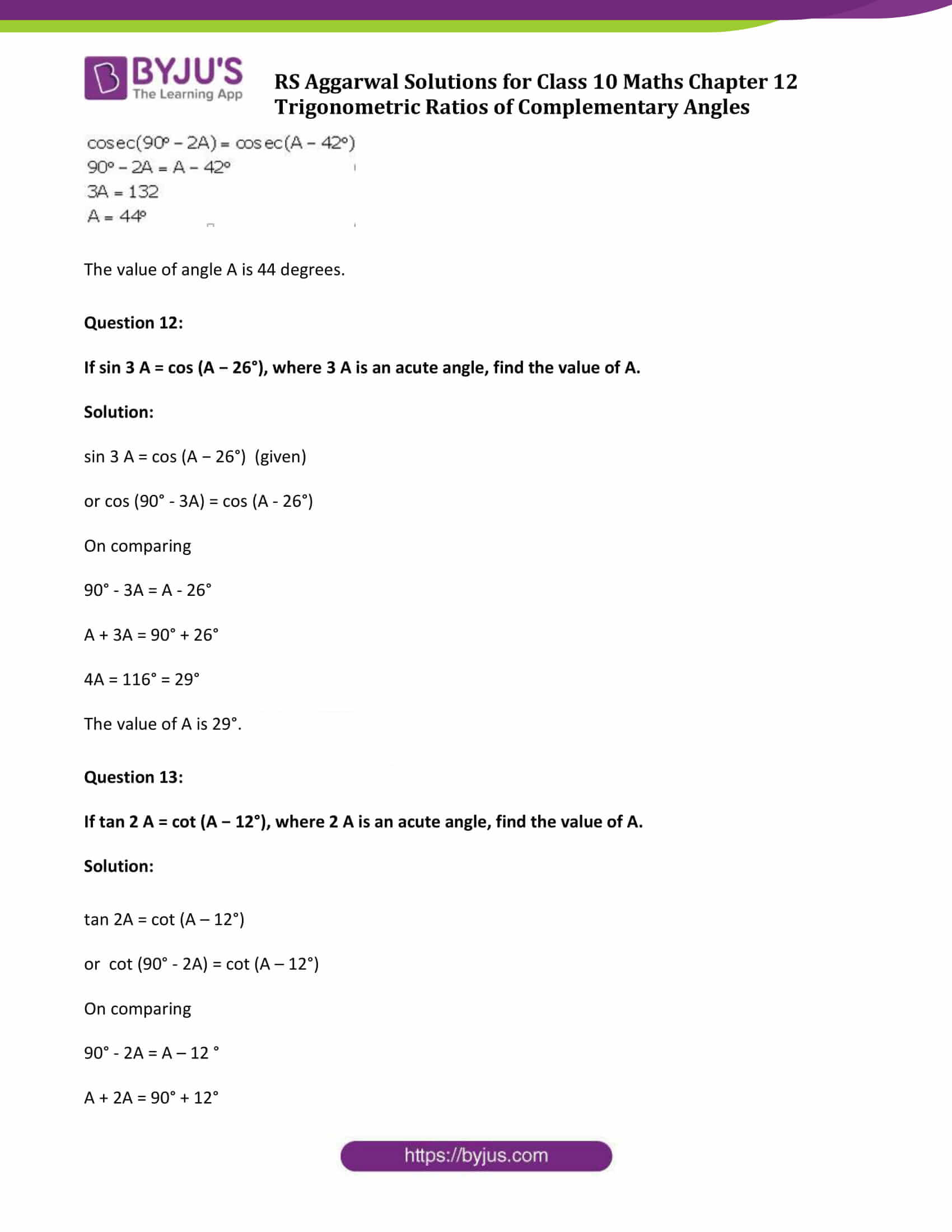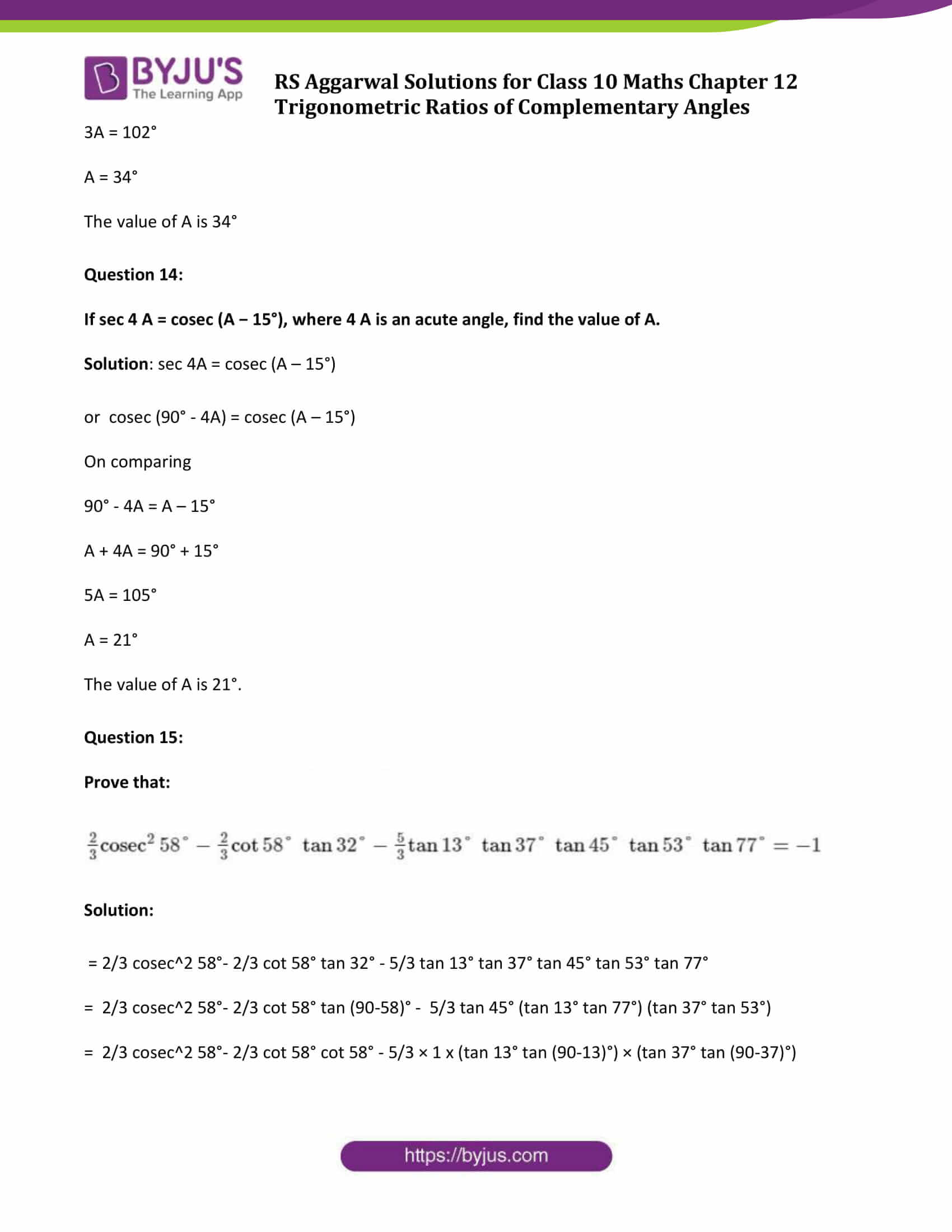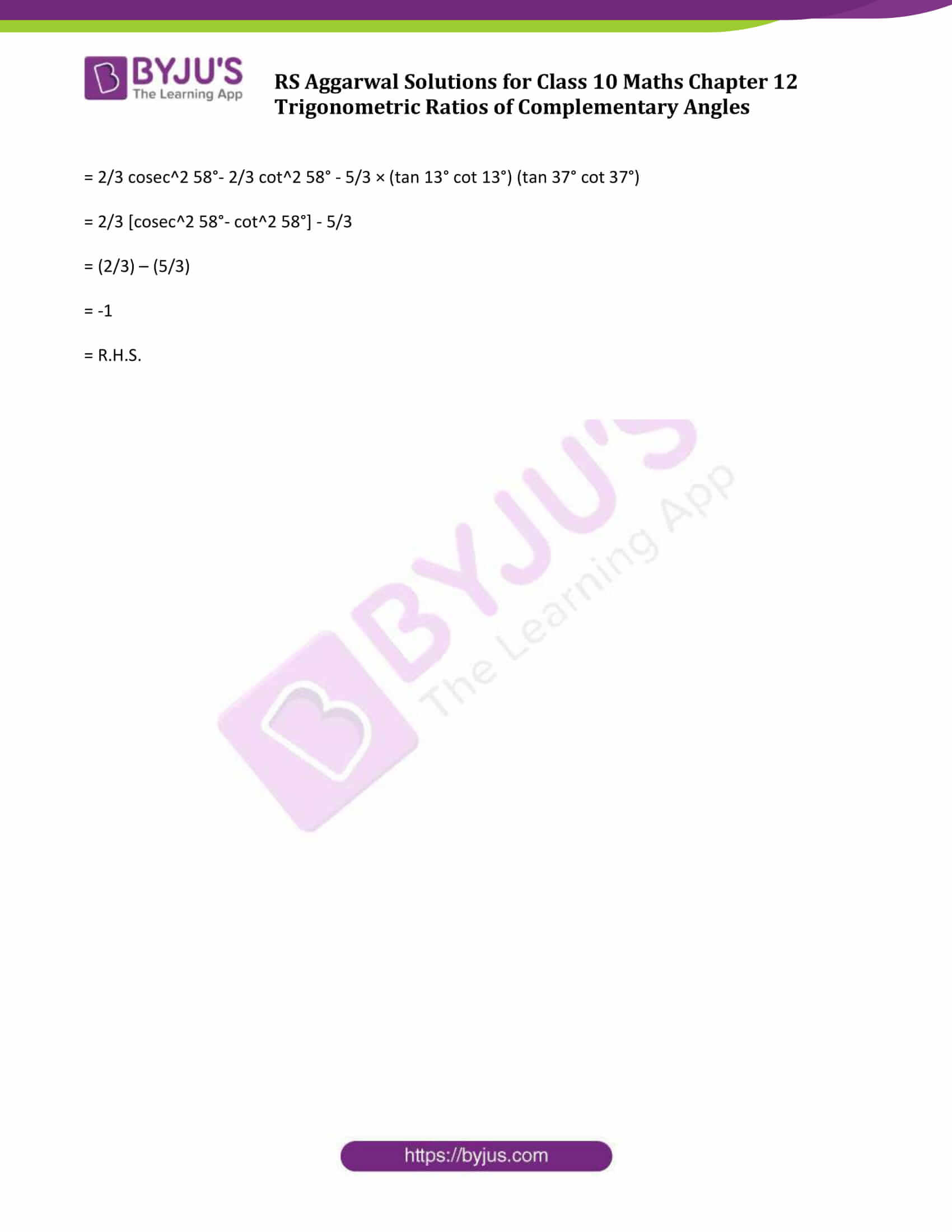## Exercise 12 Page No: 563

Question 1.

Without using trigonometric tables, evaluate: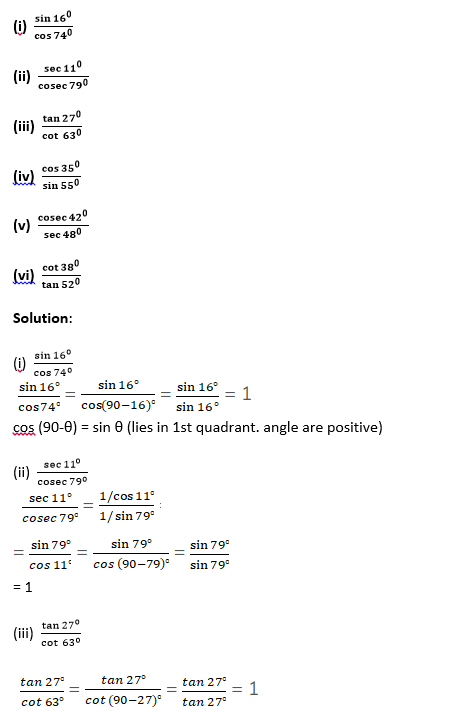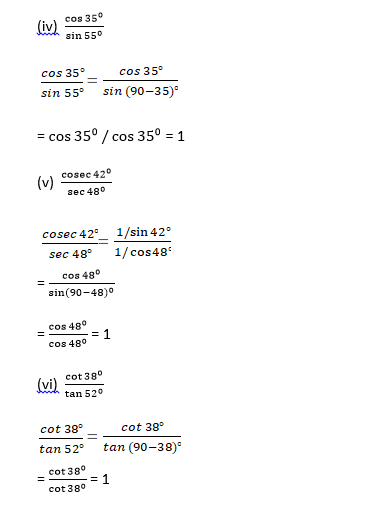Question 2:

Without using trigonometric tables, prove that:

(i) cos 81° − sin 9° = 0

(ii) tan 71° − cot 19° = 0

(iii) cosec 80° − sec 10° = 0

(iv) cosec2 72° − tan2 18° = 1

(v) cos2 75° + cos2 15° = 1

(vi) tan2 66° − cot2 24° = 0

(vii) sin2 48° + sin2 42° = 1

(viii) cos2 57° − sin2 33° = 0

(ix) (sin 65° + cos 25°)(sin 65° − cos 25°) = 0

Solution:

(i) LHS = cos81° – sin9°

= cos(90° -9°)- sin9°

= sin9° – sin9°

= 0

= RHS

(ii) LHS = tan71° – cot19°

=tan(90° – 19°) – cot19°

=cot19° – cot19°

=0

= RHS

(iii) LHS = cosec80° – sec10°

= cosec(90° – 10°) – sec(10°)

= sec10° – sec10°

= 0

= RHS

(iv) cosec^2 72° − tan^2 18° = 1

LHS = cosec^2 72° – tan^2 18°

= cosec^2 72° – tan2 (90 – 72)°

= cosec^2 72° – cot^2 72°

= 1 = RHS

(v) cos^2 75° + cos^2 15° = 1

LHS = cos^2 75° + cos^2 15°

= cos^2 75° + cos^2 (90 – 75)°

= cos^2 75° + sin^2 75°

= 1= RHS

(vi) tan^2 66° − cot^2 24° = 0

LHS = tan^2 66° − cot^2 24°

= tan^2 66° – cot^2 (90 – 66)°

= tan^2 66° – tan^2 66°

= 0

= RHS

(vii) sin^2 48° + sin^2 42° = 1

LHS = sin^2 48° + sin^2 42°

= sin^2 48° + sin^2 (90 – 48)°

= sin^2 48° + cos^2 48°

= 1

= RHS

(viii) cos^2 57° − sin^2 33° = 0

LHS = cos^2 57° − sin^2 33°

= cos^2 57° – sin^2 (90 – 57)°

= cos^2 57° – cos^2 57°

= 0

=RHS

(ix) (sin 65° + cos 25°)(sin 65° − cos 25°) = 0

LHS = (sin 65° + cos 25°)(sin 65° − cos 25°)

= sin^2 65° – cos^2 25°

= sin^2 65° – cos^2 (90 – 65)°

= sin^2 65° – sin^2 65°

= 0

=RHS

Question 3.

Without using trigonometric tables, prove that:

(i) sin 53° cos 37° + cos 53° sin37° = 1

(ii) cos 54° cos 36° − sin 54° sin36° = 0

(iii) sec 70° sin 20° + cos 20° cosec70° = 2

(iv) sin 35° sin 55° − cos 35° cos55° = 0

(v) (sin 72° + cos 18°)(sin 72° − cos18°) = 0

(vi) tan 48° tan 23° tan 42° tan67° = 1

Solution:

(i) LHS = sin53° cos37° + cos53° sin37°

= sin53° cos(90° – 53°) + cos53° sin (90° – 53°)

= sin53° x sin53° + cos53° x cos53°

= sin^2 53° + cos^2 53°

= 1

= RHS

(ii) LHS = cos54° cos36° − sin54° sin36°

= cos54° cos36° − sin(90° -36°) sin(90° -54°)

= cos54° cos36° – cos36°cos54°

= 0

=RHS

(iii) LHS = sec70° sin20° + cos20° cosec70°

= sec(90° – 20°) sin20° + cos20° cosec(90° – 20°)

= cosec 20° sin20° + cos20° sec 20°

= 1 +1

= 2

=RHS

(iv) LHS = sin35° sin55° − cos35° cos55°

= sin(90° – 55°) sin(90° – 35°) − cos35° cos55°

= cos55° cos35° – cos35° cos55°

= 0

=RHS

(v) LHS = (sin72° + cos18°)(sin72° − cos18°)

=(sin^2 72° – cos^2 18°)

= (sin^2 72° – cos^2 (90° – 72°))

= sin^2 72° – sin^2 72°

= 0

=RHS

(vi) LHS = tan48° tan23° tan42° tan67°

=tan48° tan23° tan (90° – 48°) tan (90° – 23°)

= tan48° tan23° cot48° cot23°

= 1×1

= 1

=RHS

Question 4.

Prove that: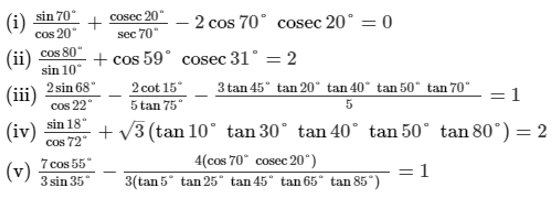Solution: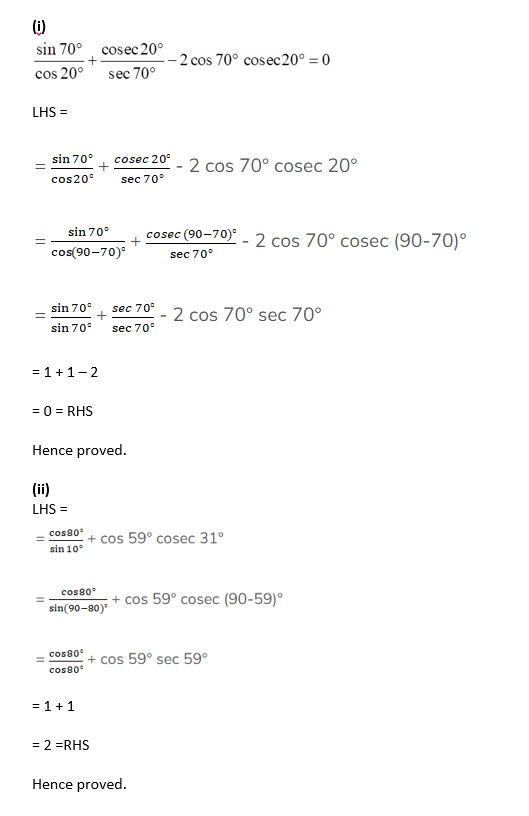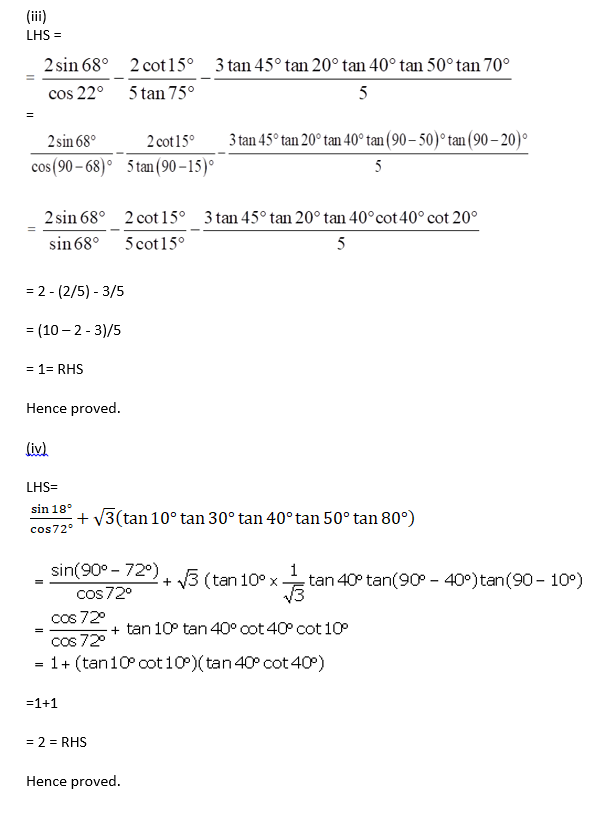(v)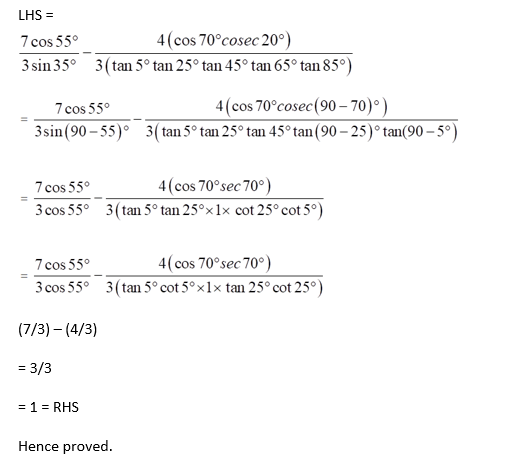Question 5.

Prove that: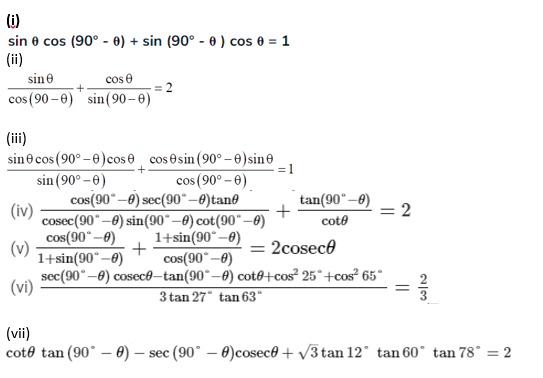Solution:

(i)

LHS = sin θ cos (90° – θ) + sin (90° – θ) cos θ

= sin θ sin θ + cos θ cos θ

= sin^2 θ + cos^2 θ

= 1

= R.H.S.

Hence proved.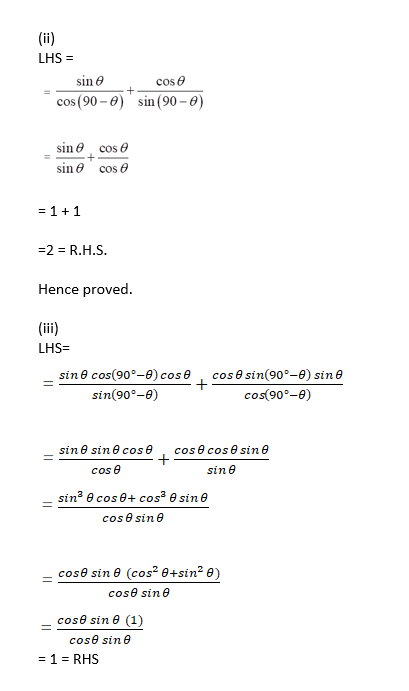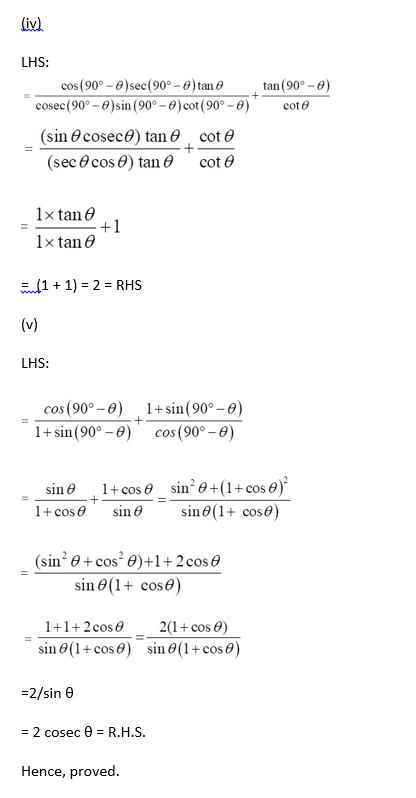(vi)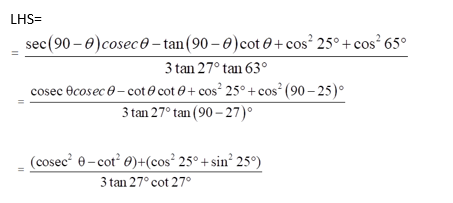=(1+1)/(3×1)

= 2/3 = RHS

Hence proved.

(vii)

LHS = cot θ tan (90° -θ) – sec (90° – θ)cosec θ +√3tan 12°tan 60°tan 78°

= cot θ cot θ – cosec θ cosec θ +√3 tan 60° tan 12° tan 78°

= cot^2 θ – cosec^2 θ +√3 tan 60° tan 12° tan(90-12)°

= – (cosec^2 θ – cot^2 θ) +√3 tan 60° tan 12° cot 12°

= – 1 + √3(√3 × 1)

= – 1 + 3

= 2

= R.H.S.

Hence proved.

Question 6:

Prove that:

(i) tan 5° tan25° tan30° tan65° tan85° = 1/√3

(ii) cot 12° cot38° cot52° cot60° cot78° = 1/√3

(iii) cos 15° cos35° cosec55° cos60° cosec75° = 1/2

(iv) cos 1° cos2° cos3° … cos180° = 0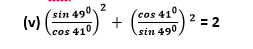Solution: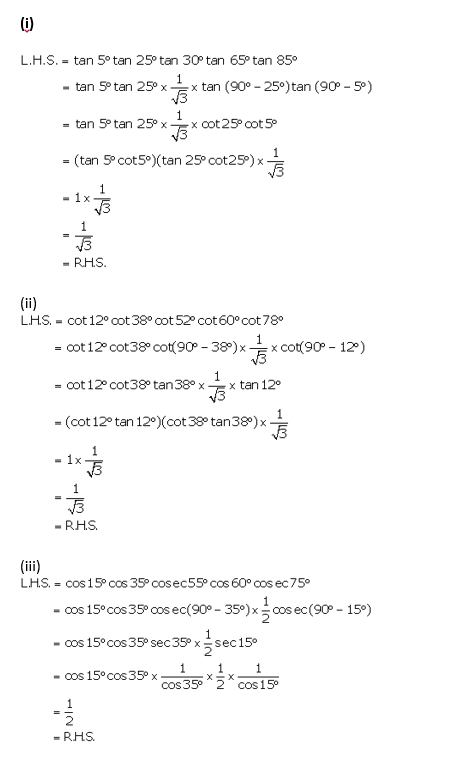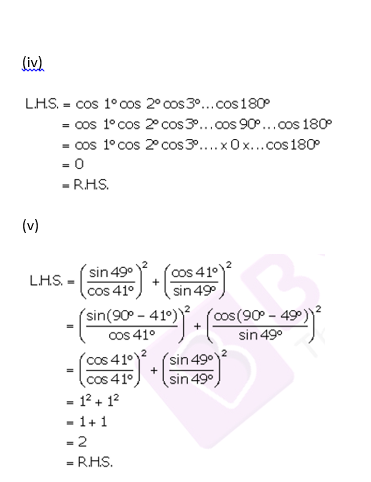Question 7:

Prove that

(i) sin (70° + θ) − cos (20° − θ) = 0

(ii) tan (55° − θ) − cot (35° + θ) = 0

(iii) cosec (67° + θ) − sec (23° − θ) = 0

(iv) cosec (65 °+ θ) sec (25° − θ) − tan (55° − θ) + cot (35° + θ) = 0

(v) sin (50° + θ ) − cos (40° − θ) + tan 1° tan 10° tan 80° tan 89° = 1.

Solution:

L.H.S.

= sin (70°+θ) — cos (20° — θ)

= sin (70°+θ) — cos [90°-(70° + θ)]

= sin (70°+θ) — sin (70° + θ)

= 0

= R.H.S.

Hence Proved.

(ii)

L.H.S.

= tan (55° — θ) — cot (35° + θ)

= tan (90°-(35° +θ)) — cot (35° + θ)

= cot (35° + θ) – cot (35° + θ)

= 0

=RHS

Hence Proved.

(iii)

L.H.S.

= cosec (67° + θ) — sec (23° — θ)

= cosec (67° + θ) – sec (90° —(23° + θ))

= cosec (67° + θ) – cosec (67° + θ)

= 0

=RHS

Hence Proved.

(iv)

L.H.S.

= cosec (65° + θ) — sec (25° — θ) — tan (55° — θ) + cot (35° + θ)

= cosec (65° + θ) — sec (90° – (65° + θ)) — tan (90° – (35° + θ)) + cot (35° + θ)

= cosec (65° + θ) — cosec (65° + θ)) — cot (35° + θ) + cot (35° + θ)

= 0

= R.H.S.

(v)

L.H.S.

= sin (50° +θ) — cos (40° — θ) + tan 1° tan 10° tan 80° tan 89°

= sin ((90°-(40° – θ)) — cos (40° — θ) + (tan 1° tan 89°)(tan10° tan 80°)

= cos (40° – θ) – cos (40° – θ) + {tan 1° tan (90°-1°)}{tan10° tan(90°-10°)}

= 0 + {(tan 1° cot 1°}{tan10° cot 10°}

= 0 + 1 = 1

= R.H.S.

Question 8.

Express each of the following in terms of trigonometric ratios of angles lying between 0° and 45°.

(i) sin 67° + cos 75°

(ii) cot 65° + tan49°

(iii) sec78° + cosec56°

(iv) cosec54° + sin72°

Solution: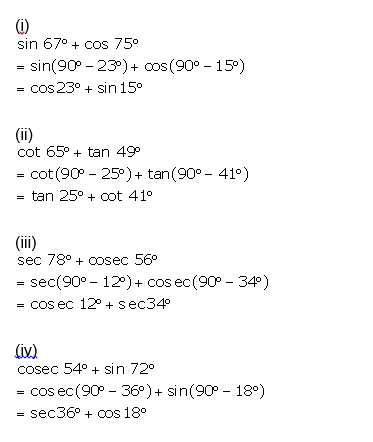Question 9.

If A, B and C are the angles of a ∆ABC, prove that

$tan\frac{C+A}{2} = cot\frac{B}{2}$.

Solution:

Given function is : $tan\frac{C+A}{2} = cot\frac{B}{2}$

Sum of all the angles of a triangle = 180 degree

So, A + B + C = 180o

Or A + C = 180o – B

And, (A + C)/2 = (180o – B)/2 = 90o – B/2

Now, tan (A + C)/2 = tan(90o – B/2) = cot B/2

Hence Proved.

Question 10.

If cos2θ = sin4θ, where 2θ and 4θ are acute angles, then find the value of θ.

Solution:

cos 2θ = sin 4θ …(1)

We know that,

sin( 90° – θ) = cos θ

So, equation (1) can be written as

sin (90° – 2θ) = sin 4θ

On comparing both sides

90° – 2θ = 4θ

90° = 4θ + 2θ

6θ = 90°

or θ = 15°

The value of θ is 15°

Question 11: If sec2A = cosec(A − 42°), where 2A is an acute angle, then find the value of A.

Solution: Given: sec2A = cosec(A − 42°)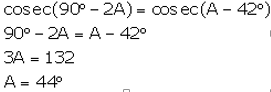The value of angle A is 44 degrees.

Question 12:

If sin 3 A = cos (A − 26°), where 3 A is an acute angle, find the value of A.

Solution:

sin 3 A = cos (A − 26°) (given)

or cos (90° – 3A) = cos (A – 26°)

On comparing

90° – 3A = A – 26°

A + 3A = 90° + 26°

4A = 116° = 29°

The value of A is 29°.

Question 13:

If tan 2 A = cot (A − 12°), where 2 A is an acute angle, find the value of A.

Solution:

tan 2A = cot (A – 12°)

or cot (90° – 2A) = cot (A – 12°)

On comparing

90° – 2A = A – 12 °

A + 2A = 90° + 12°

3A = 102°

A = 34°

The value of A is 34°

Question 14:

If sec 4 A = cosec (A − 15°), where 4 A is an acute angle, find the value of A.

Solution: sec 4A = cosec (A – 15°)

or cosec (90° – 4A) = cosec (A – 15°)

On comparing

90° – 4A = A – 15°

A + 4A = 90° + 15°

5A = 105°

A = 21°

The value of A is 21°.

Question 15:

Prove that: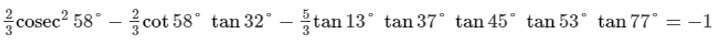Solution:

= 2/3 cosec^2 58°- 2/3 cot 58° tan 32° – 5/3 tan 13° tan 37° tan 45° tan 53° tan 77°

= 2/3 cosec^2 58°- 2/3 cot 58° tan (90-58)° – 5/3 tan 45° (tan 13° tan 77°) (tan 37° tan 53°)

= 2/3 cosec^2 58°- 2/3 cot 58° cot 58° – 5/3 × 1 x (tan 13° tan (90-13)°) × (tan 37° tan (90-37)°)

= 2/3 cosec^2 58°- 2/3 cot^2 58° – 5/3 × (tan 13° cot 13°) (tan 37° cot 37°)

= 2/3 [cosec^2 58°- cot^2 58°] – 5/3

= (2/3) – (5/3)

= -1

= R.H.S.

## R S Aggarwal Solutions for Chapter 12 Trigonometric Ratios of Complementary Angles Topics

In this chapter students will study important concepts on the topic as listed below:

• Complementary angles introduction.

Two angles are complementary angles if their sum is 90 degrees. For example, if one angle is x^o then second angle will be (90-x)^o.

• Learn to evaluate various trigonometric ratios without using trigonometric tables.

RS Aggarwal Solutions for Class 10 Chapter 12 Trigonometric ratios of complementary angles provides step by step answers, which help students understand the concepts easily. Download and practice R S Aggarwal Solutions for Maths to score more in your exams.

### Key Features of R S Aggarwal Solutions for Class 10 Maths Chapter 12 Trigonometric ratios of Complementary Angles

1. R S Aggarwal provides conceptual knowledge on the topic trigonometric ratios of complementary angles.

2. Helps to crack competitive exams.

3. Set of various problems along with their answers.

4. All questions are answered step by step by subject experts.